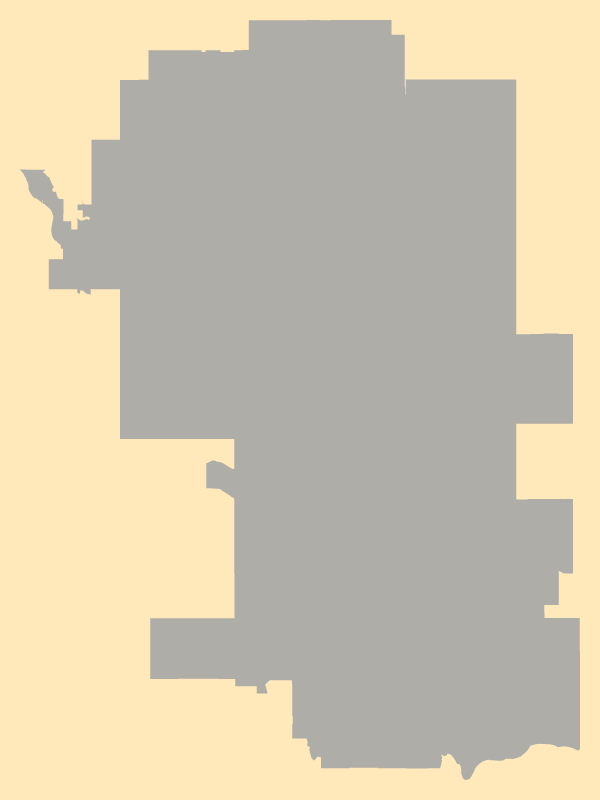Although using mapping application like Google Maps, Mapbox or Leaflet can be extremely useful, I was looking for a way to draw simple GeoJSON files in my Processing/p5.js sketches. I didn’t want to use a library to integrate my GeoJSON files and I wanted more flexibility in how my maps could be drawn and manipulated. I came across this post by Mike Fowler that achieved exactly what I was considering so I went about implementing this method in p5.js.

I used the city boundary GeoJSON data available from the City of Calgary’s Open data catalogue.

The getBoundingBox algorithm is almost exactly the same as Mike Fowler’s:

function getBoundingBox (boundary) {

let coords,latitude, longitude;
let data = boundary.the_geom.coordinates;

for (var i = 0; i < data.length; i++) {
coords = data[i];

for (var j = 0; j < coords.length; j++) {
longitude = coords;
latitude = coords;
bounds.xMin = bounds.xMin < longitude ? bounds.xMin : longitude; bounds.xMax = bounds.xMax > longitude ? bounds.xMax : longitude;
bounds.yMin = bounds.yMin < latitude ? bounds.yMin : latitude; bounds.yMax = bounds.yMax > latitude ? bounds.yMax : latitude;
}
}
return bounds;
}


The P5.js  Map() function allowed me to be quickly map the latitude and longitude values to the canvas width and height using the bounding box values determined above. From there, I simply created a shape using the Shape() function:

function draw() {

let data = boundary.the_geom.coordinates;
noStroke();
fill(25,45,78);
beginShape();
for (var i = 0; i < data.length; i++) {
let lon = boundary.the_geom.coordinates[i];
let lat = boundary.the_geom.coordinates[i];